## RD Sharma Class 10 Solutions Chapter 2 Polynomials Ex 2.2

These Solutions are part of RD Sharma Class 10 Solutions. Here we have given RD Sharma Class 10 Solutions Chapter 2 Polynomials Ex 2.2

Other Exercises

Question 1.
Verify that numbers given along side of the cubic polynomials below are their zeros. Also, verify the relationship between the zeros and coefficients in each case :
(i) f(x) = 2x3 – x2 – 5x + 2 ; $$\frac { 1 }{ 2 }$$ , 1, -2
(ii) g(x) = x3 – 4x2 + 5x – 2 ; 2, 1, 1
Solution: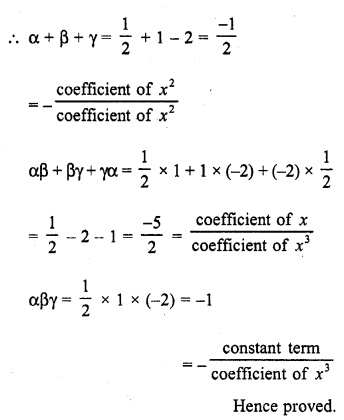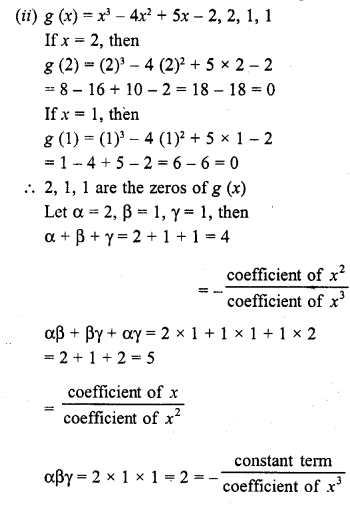Question 2.
Find a cubic polynomial with the sum, sum of product of its zeros taken two at a time and product of its zeros as 3, -1 and -3 respectively.
Solution:Question 3.
If the zeros of the polynomial f(x) = 2x3 – 15x2 + 37x – 30 are in AP. Find them.
Solution: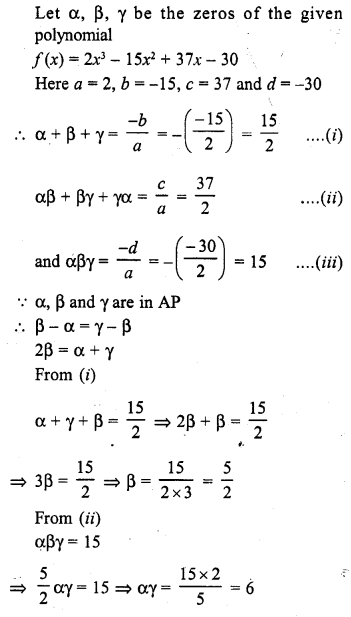Question 4.
Find the condition that the zeros of the polynomial f(x) = x3 + 3px2 + 3qx + r may be in AP.
Solution:Question 5.
If the zeros of the polynomial f(x) = ax3 + 3bx2 + 3cx + d are in A.P., prove that 2b3 – 3abc + a2d = 0.
Solution: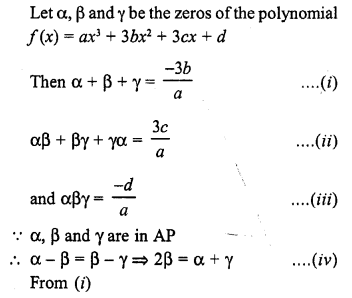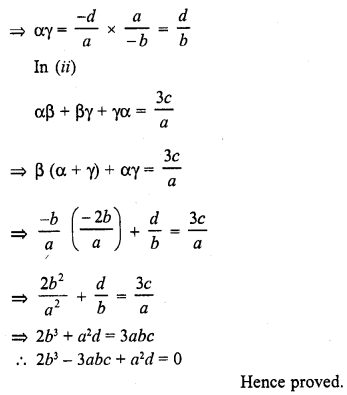Question 6.
If the zeros of the polynomial f(x) = x3 – 12x2 + 39x + k are in AP, find the value of k.
Solution: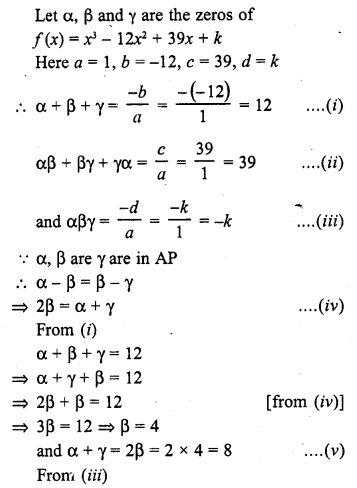Hope given RD Sharma Class 10 Solutions Chapter 2 Polynomials Ex 2.2 are helpful to complete your math homework.

If you have any doubts, please comment below. Learn Insta try to provide online math tutoring for you.